## Sunday, June 17, 2012

### The Fifth Degree: Part 1

I'm kind of stalled on physics these days, so maybe this would be a good time to catch up on some math topics. Let's see where this one goes...

One of the most mind-boggling acheivements in the history of human thought is the proof that the fifth degree equation is unsolvable. It's one thing to solve a hard equation, and another thing to prove that you can't solve it. It's a fascinating story that isn't well told.

We torture high school students with the solution of the quadratic equation. Most people will remember it as the hardest formula they ever had to memorize. This completely misses the essential point about the solving the quadratic: the equation is the simplest possible equation that makes sense. Reduced to its essence, the quadratic formula basically says: the solution of the quadratic equations is a number plus the square root of a number: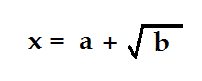It's a flawless formula because the number b has two square roots, and there are two solutions of the quadratic formula. Calling them alpha and beta, we can write: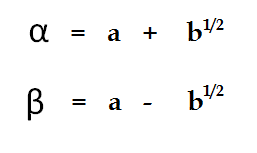The real beauty of the formula is that it doesn't ask you to distinguish between the positive and negative square roots. They are both correct, and they both give you solutions to the same equation. Incidentally, it's amazing how easy it is to solve a quadratic equation using this reasoning as compared to the univerally-taught method of "completing the square" that you remember from high school. You just have to remember understand that the coefficients of the quadratic equation are actually the sum and product of the roots: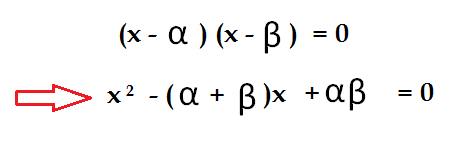The sum and product of alpha and beta are called the elementary symmetric polynomials in alpha and beta, and they have very simple expressions in terms of our a and b, those as-yet-undetermined numbers in which the roots will ultimately be expressed: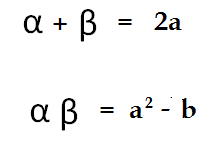Do you see that if we simply identify these last two expressions with the coefficients of the quadratic as expanded in the previous equation, that the quadratic solves amost effortlessly?

A fascinating thing happens when we move on to the cubic equation. Here again, the standard treatments are horribly misguided, by my way of thinking. Normally they take you through some very difficult algebra whose end result is a horribly intimidating mass of symbols. "This", they tell you, "is the solution of the cubic equation. The fourth degree is even more complicated."

Instead of emphasizing the complexity of the solutions, the correct approach is to recognize their simplicity. We look for the simplest possible solution of the cubic equation: and by analogy with our simplified solution to the quadratic equation, we look first to the obvious: a number plus the cube root of a number: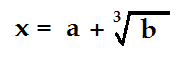This is our trial solution, and a few moments reflection is sufficient to show it doesn't do the job. It starts of well: a cubic equation must have three solutions, and since the number b has three cube roots...so far, so good. Taking omega as a cube root of unity, we can even write the three roots out explicitly, as we did with the quadratic: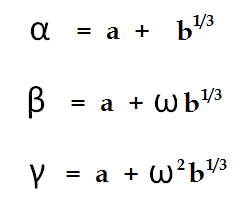It's a nice formula that takes on exactly three values...note that it doesn't matter if we take b to be a real or complex cube root, we get the same three values for alpha beta and gamma no matter what choice we make. But is soon becomes apparent that this simple formula just doesn't have enough versatility to take care of all the things a cubic equation can do. For one thing, it obviously can't generate three real values, which rules out all kinds of perfectly good equations. We need something more complicated.

In particular, since every quadratic equation is also a cubic equation (albeit with a leading coefficient of zero), we need a formula that allows for taking square roots as well as cube roots. The least complicated formulas that fill these minimal requirements would be something like this: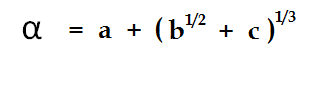Or perhaps something similar with the cube root inside the brackets instead of the square root. Either way, we are at least making progress. But there is something horribly wrong with this proposed solution. Where our previous guess was not powerful enough to do the job, this one is too powerful. Or at least, it generates too many solutions. With our quadratic equation, we had a formula that generated two solutions, which was just what we needed. Now we have a formula that generates six solutions: two choices for the square root, and three choices for the cube root. That's twice as many solutions as we want. It's not a good sign.

In fact, it's not too hard to verify that formula we've written is the solution of a fairly simple sixth-degree equation in alpha. You can generate the exact equation by cubing both sides, collecting terms, and then squaring both sides again. But that's not where we want to go with this.

The real question is: how do we write a solution for the cubic equation that is powerful enough to do the job, and at the same time doesn't generate too many solutions?

Let's take that up when we return.

Money Worksheets said...

This blog is full of knowledge and facts.Physics is a study of real life phenomenons with help of mathematics.

Marty Green said...

I think you're telling me not to apologise for doing math instead of physics, and I appreciate that. I have to admit, however, that this is a topic that doesn't have much connection to anything physical. Although there are people who make a big deal of group theory, which is a big part of the present story.